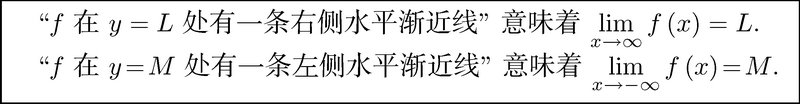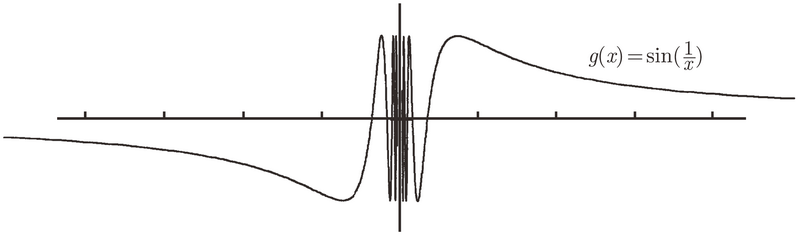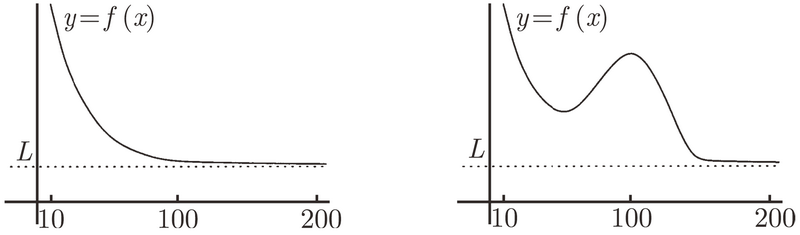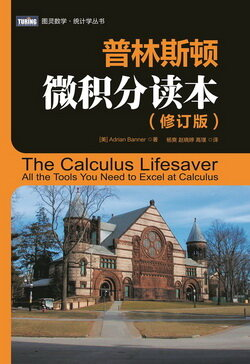# 普林斯顿微积分读本（修订版）(24)：极限导论 3.4(在 ∞ 和 -∞ 处的极限)

$\lim_{x\to\infty}f(x)=L,$

$\lim_{x\to-\infty}f(x)=L,$1 如果你不信, 请参见附录 A 的 A.4.1 节!

$\lim_{x\to\infty}\sin(1/x)=0.$

$f(-x)=\sin\biggl(\frac{1}{-x}\biggr)=\sin\biggl(-\frac{1}{x}\biggr)=-\sin\biggl(\frac{1}{x}\biggr)=-f(x).$• 如果一个数的绝对值是非常大的数, 则这个数是大的;
• 如果一个数非常接近于 0(但不是真的等于 0), 则这个数是小的.

$\lim_{x\to\infty}f(x)=L.$$\lim_{x\to0}f(x)=L,\quad\lim_{x\to0^+}f(x)=L$　或　$\lim_{x\to0^-}f(x)=L$.